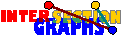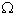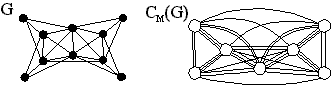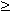# Intersection Multigraphs

In the intersection multigraphM(H) of a hypergraph H=(A,(S_x/xV)), distinct vertices x,y are connected by |SxSy| parallel edges. As t-intersection, it mainly makes sense in discrete models. Intersection multigraphs contain all the information of all t-intersection graphs.

We shall see in the following subsection how intersection multigraphs of the cliques have some use for other, ordinary intersection graph models.

### Clique Multigraphs

There is another way of finding out which cliques are star graphs in line graphs. Although not really necessary there, it becomes necessary for other models presented later. We have to define the clique multigraph CM(G) of G. This is just the intersection multigraph of the set of all cliques of G, i.e. the cliques of G are the vertices of CM(G), and two vertices are joined by as many parallel edges as the cliques have vertices in common. Here is an example:Now observe that for line graphs G the number of edges between every pair of adjacent vertices in CM(G) reveals whether both have the same type---star graph or not. Two star graphs have at most one common vertex. Two non star graph cliques must stem from different triangles, and therefore have also at most one common vertex. But a star graph and a non star graph have either none or two common vertices. Since CM(G) is connected for connected G, the vertices of CM(G) should partition into two sets, one of which should give all star graph cliques.

We will give three more examples where the clique multigraph plays an important role for the recognition problem.

The first use of clique multigraphs in intersection graph theory is the following theorem, which is due to Bernstein and Goodman, which answers the reconstruction problem for chordal graphs completely.[BG81]: A chordal graph G is the intersection graph of subtrees of some tree T if and only if T is contractible to some maximum spanning tree of the clique multigraph CM(G) of G. Proof:

Consider the version of Example 8 where we do not know how many species have both two given features, but simply whether there are such species at all. Then we only have the intersection information of the features. We may apply the theorem and get at least some model, see the right Figure below. When we ask whether some particular tree could be the model, however, the Theorem might be of less use. Actually, the following problem is NP-complete.

• Instance: A tree T and some weighted graph W, both with the same number of vertices.
• Question: Is some maximum spanning tree of W isomorphic to T?
For instance, if the tree is a path, and if all edge weights are equal, this is just the problem of finding a Hamiltonian path, if one exists.What is the complexity of the above problem restricted to clique multigraph of chordal graphs?Is there a good characterization of clique multigraphs of chordal graphs?

### For its own sake

So it is presently unknown how to recognize clique multigraphs of chordal graphs, whereas clique graphs of chordal graphs are efficiently recognizable. Here I will present an example for the contrary effect, where intersection multigraphs seem easier to recognize. Let us start with so-called chordal multigraphs, intersection multigraphs of subtrees of some tree. This essentially geometrical model could also be interpreted as discrete, and in many applications, trees are rather discrete than continuous, and then the notion of intersection multigraphs fits.

For an edge xy in some multigraph, let m(xy) denote the number of parallel edges from x to y, and let k(xy) denote the number of cliques of the underlying graph containing edge xy.[Mc91]: A multigraph is chordal if and only if its underlying graph is chordal and it fulfills the mk condition. Proof:By a construction like in the proof, one gets the representation (phylogenetic tree) (to the left) of the example multigraph. On the right is just a ordinary intersection representation of the underlying graph. Compared with this, in the multigraph model we detect that there must be one more (hidden) species having features A and H.

A similiar, but slightly more complicated, characterization of so-called interval multigraphs---intersection multigraphs of subpaths of some paths--- has also been given by McKee.Is there some multigraph variant of Bernstein and Goodman´s. Theorem? How could one decide whether a given chordal multigraph is representable on some given tree?

Whereas it is still not known how th recognize k-line graphs, the multigraph version, intersection multigraphs of 3-uniform 3-conformal hypergraphs, can be recognized in polynomial time, by the standard approach of first determining all star graphs (with respect to 2-intersection) and then putting pieces together [P98]. Still, without 3-conformality, it is unknown.

Back to the start page for intersection graphs.

Erich Prisner